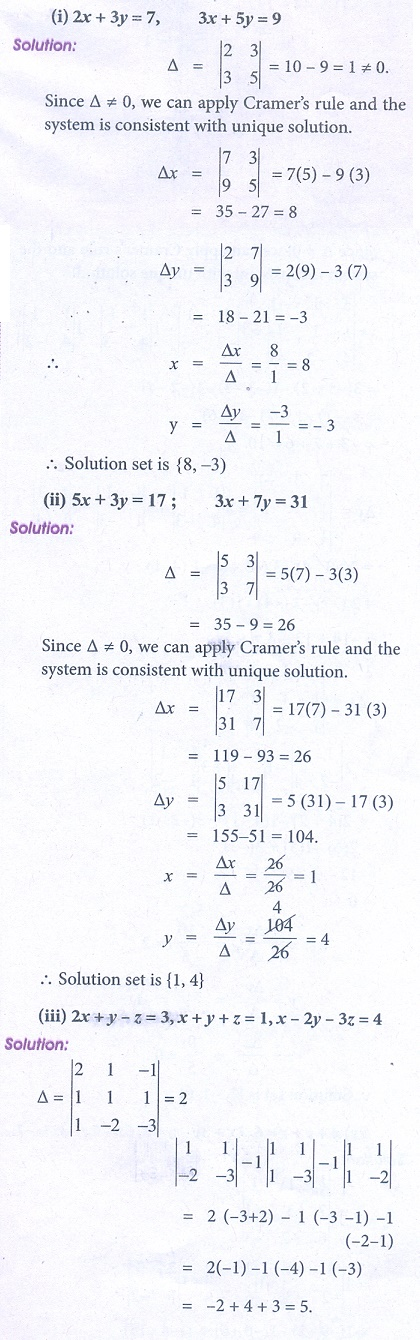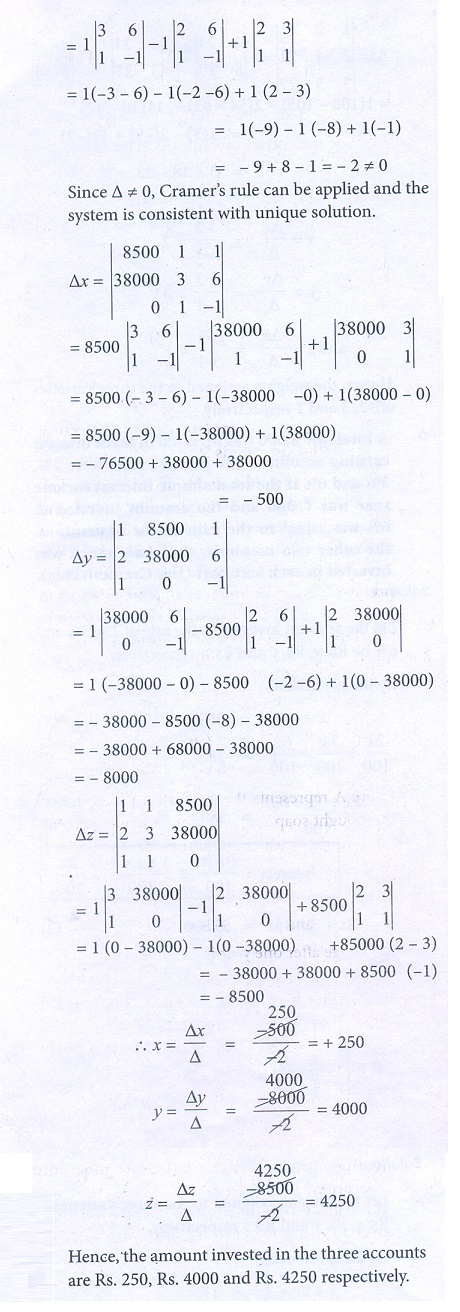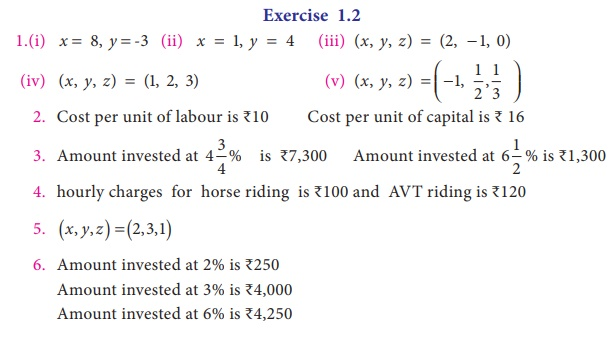Home | | Business Maths 12th Std | Exercise 1.2 : CramerŌĆÖs Rule

# Exercise 1.2 : CramerŌĆÖs Rule

Book back answers and solution for Exercise questions - Business Maths and Statistics : Applications of Matrices and Determinants: CramerŌĆÖs Rule

Exercise 1.2

1. Solve the following equations by using CramerŌĆÖs rule

(i) 2x + 3y = 7; 3x + 5y = 9

(ii) 5x + 3y = 17; 3x + 7 y = 31

(iii) 2x + y ŌłÆ z = 3, x + y + z = 1, x ŌłÆ 2 y ŌłÆ 3z = 4

(iv) x + y + z = 6, 2x + 3y ŌłÆ z = 5, 6x ŌłÆ 2 y ŌłÆ 3z = ŌłÆ 7

(v) x + 4 y + 3z = 2, 2x ŌłÆ 6 y + 6z = ŌłÆ3, 5x ŌłÆ 2 y + 3z = ŌłÆ52. A commodity was produced by using 3 units of labour and 2 units of capital, the total cost is Ōé╣62. If the commodity had been produced by using 4 units of labour and one unit of capital, the cost is Ōé╣56. What is the cost per unit of labour and capital? (Use determinant method).3. A total of Ōé╣8,600 was invested in two accounts. One account earned 4 3/4 % annual1 interest and the other earned 6 1/2 % annual interest. If the total interest for one year was Ōé╣431.25, how much was invested in each account? (Use determinant method).4. At marina two types of games viz., Horse riding and Quad Bikes riding are available on hourly rent. Keren and Benita spent Ōé╣780 and Ōé╣560 during the month of May.Find the hourly charges for the two games (rides). (Use determinant method).5. In a market survey three commodities A, B and C were considered. In finding out the index number some fixed weights were assigned to the three varieties in each of the commodities. The table below provides the information regarding the consumption of three commodities according to the three varieties and also the total weight received by the commodityFind the weights assigned to the three varieties by using CramerŌĆÖs Rule.6. A total of Ōé╣8,500 was invested in three interest earning accounts. The interest rates were 2%, 3% and 6% if the total simple interest for one year was Ōé╣380 and the amount invested at 6% was equal to the sum of the amounts in the other two accounts, then how much was invested in each account? (use CramerŌĆÖs rule).